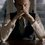# Binomial Coefficient Challenge 12!

Prove that

$\displaystyle \sum_{k=0}^{m-1}{\frac{{(-1)}^k}{\binom{n}{k}} H_k} = \frac{n+1}{n+2}\left(\frac{{(-1)}^{m+1}}{\binom{n+1}{m}}\left(H_m - \frac{1}{n+2}\right) - \frac{1}{n+2}\right)$

where $H_m$ is the $m$th Harmonic number.

PS - This is what I am getting. If you get something else, you can report.Note by Kartik Sharma
4 years, 1 month ago

This discussion board is a place to discuss our Daily Challenges and the math and science related to those challenges. Explanations are more than just a solution — they should explain the steps and thinking strategies that you used to obtain the solution. Comments should further the discussion of math and science.

When posting on Brilliant:

• Use the emojis to react to an explanation, whether you're congratulating a job well done , or just really confused .
• Ask specific questions about the challenge or the steps in somebody's explanation. Well-posed questions can add a lot to the discussion, but posting "I don't understand!" doesn't help anyone.
• Try to contribute something new to the discussion, whether it is an extension, generalization or other idea related to the challenge.

MarkdownAppears as
*italics* or _italics_ italics
**bold** or __bold__ bold
- bulleted- list
• bulleted
• list
1. numbered2. list
1. numbered
2. list
Note: you must add a full line of space before and after lists for them to show up correctly
paragraph 1paragraph 2

paragraph 1

paragraph 2

[example link](https://brilliant.org)example link
> This is a quote
This is a quote
    # I indented these lines
# 4 spaces, and now they show
# up as a code block.

print "hello world"
# I indented these lines
# 4 spaces, and now they show
# up as a code block.

print "hello world"
MathAppears as
Remember to wrap math in $$ ... $$ or $ ... $ to ensure proper formatting.
2 \times 3 $2 \times 3$
2^{34} $2^{34}$
a_{i-1} $a_{i-1}$
\frac{2}{3} $\frac{2}{3}$
\sqrt{2} $\sqrt{2}$
\sum_{i=1}^3 $\sum_{i=1}^3$
\sin \theta $\sin \theta$
\boxed{123} $\boxed{123}$

Sort by:

We have,

$\text{S} = \sum_{k=0}^{m-1} \dfrac{{(-1)}^k}{\dbinom{n}{k}} H_k = \sum_{k=1}^{m-1} \dfrac{{(-1)}^k}{\dbinom{n}{k}} H_k$

$= \sum_{r=1}^{m-1} \sum_{k=r}^{m-1} \dfrac{1}{r} \dfrac{{(-1)}^k}{\binom{n}{k}}$

$= \sum_{r=1}^{m-1} \dfrac{1}{r} \left[ \sum_{k=0}^{m-1} \left( \dfrac{{(-1)}^k}{\binom{n}{k}} \right) - \sum_{k=0}^{r-1} \left(\dfrac{{(-1)}^k}{\binom{n}{k}} \right) \right]$

$= \sum_{r=1}^{m-1} \dfrac{1}{r} (F(m) - F(r))$

$F(m) = \sum_{k=0}^{m-1} \left( \dfrac{{(-1)}^k}{\binom{n}{k}} \right) = \left(\dfrac{n+1}{n+2}\right) \left(1+\dfrac{(-1)^{m+1}}{\dbinom{n+1}{m}}\right)$

$\implies \text{S} = \left( \dfrac{n+1}{n+2} \right) \sum_{r=1}^{m-1} \dfrac{1}{r} \left[ \dfrac{(-1)^{m+1}}{\dbinom{n+1}{m}} - \dfrac{(-1)^{r+1}}{\dbinom{n+1}{r}} \right]$

$= \left( \dfrac{n+1}{n+2} \right) \left[ \dfrac{(-1)^{m+1}}{\dbinom{n+1}{m}} H_{m-1} - \sum_{r=1}^{m-1} \dfrac{(-1)^{r+1}}{r \dbinom{n+1}{r}} \right]$

$= \left( \dfrac{n+1}{n+2} \right) \left[ \dfrac{(-1)^{m+1}}{\dbinom{n+1}{m}} H_{m-1} - \dfrac{1}{n+1} \sum_{r=0}^{m-2} \dfrac{(-1)^{r}}{ \dbinom{n}{r}} \right]$

$= \left( \dfrac{n+1}{n+2} \right) \left[ \dfrac{(-1)^{m+1}}{\dbinom{n+1}{m}} H_{m-1} - \dfrac{1}{n+2} \left( 1 + \dfrac{(-1)^m}{\dbinom{n+1}{m-1}} \right) \right]$

Simplifying, we get,

$\sum_{k=0}^{m-1} \dfrac{{(-1)}^k}{\dbinom{n}{k}} H_k = \left(\dfrac{n+1}{n+2} \right)\left( \dfrac{(-1)^{m+1}}{\dbinom{n+1}{m}} \left( H_{m} - \dfrac{1}{n+2} \right) - \dfrac{1}{n+2} \right) \quad \square$

- 4 years, 1 month ago

The following editing may feel weird if you are seeing it for the first time.

We will use the fact that the finite sum -

$\displaystyle \sum {\frac{{(-1)}^k}{\binom{n}{k}} \delta k} = \frac{n+1}{n+2} \frac{{(-1)}^{k+1}}{\binom{n+1}{k}}$

This is proven here in BCC 6. A finite sum is just like indefinite integral, it must come with a constant but we are going to bound it eventually(where constants will cancel).

Or,

$\displaystyle \Delta\left(\frac{n+1}{n+2} \frac{{(-1)}^{k+1}}{\binom{n+1}{k}}\right) = \frac{{(-1)}^k}{\binom{n}{k}}$

Let

$\displaystyle S = \sum {\frac{{(-1)}^k}{\binom{n}{k}} H_k \delta k}$

Now, we use Summation By Parts -

$\displaystyle \sum {u(x) \Delta(v(x))} = u(x)v(x) - \sum {v(x+1) \Delta(u(x)}$

where $\Delta(a(x)) = a(x+1) - a(x)$.

For the current sum, take $u(k) = H_k, v(k) = \frac{n+1}{n+2} \frac{{(-1)}^{k+1}}{\binom{n+1}{k}}$.

Then,

$\displaystyle S = \frac{n+1}{n+2} \frac{{(-1)}^{k+1}}{\binom{n+1}{k}} H_k - \sum {\frac{n+1}{n+2} \frac{{(-1)}^{k+2}}{\binom{n+1}{k+1}} \frac{\delta k }{k+1}}$

$\displaystyle = \frac{n+1}{n+2} \frac{{(-1)}^{k+1}}{\binom{n+1}{k}} H_k - \sum {\frac{1}{n+2} \frac{{(-1)}^{k}}{\binom{n}{k}} \delta k}$

$\displaystyle = \frac{n+1}{n+2} \frac{{(-1)}^{k+1}}{\binom{n+1}{k}} H_k - \frac{n+1}{{(n+2)}^2} \frac{{(-1)}^{k+1}}{\binom{n+1}{k}}$

Now, we can put our bounds $0$ and $m$ (yes, not $m-1$)

$\displaystyle = \frac{n+1}{n+2}\left(\frac{{(-1)}^{m+1}}{\binom{n+1}{m}}\left(H_m - \frac{1}{n+2}\right) - \frac{1}{n+2}\right)$

PS - If you think I am cheating, then I must tell you that this method may not look like clever, but the theorems of finite calculus I have used(without mentioning) is just basic algebra. It simplifies many sums by cutting the repetitive tasks we would do for each sum. If you feel interested, grab a book on finite calculus.

- 4 years, 1 month ago

- 4 years, 1 month ago

Check the limits on the LHS - there is no $H_0$.

Is there any relationship between $m$ and $n$, or is this general? I can see a possible way through this one, but want to be sure as to the problem before I start...

- 4 years, 1 month ago

Exactly. There was this problem with $H_0$. I am not sure what the author is considering it. I have considered it to be zero. It doesn't really show much in the working anyway.

Yes. There is no relation between $m$ and $n$.

- 4 years, 1 month ago

$H_{0}$ is generally defined to be $0$. One way to look at it is to use the integral definition,

$H_{n} = \int_{0}^{1} \dfrac{x^n - 1}{x-1} \ \mathrm{d}x$

and put $n=0$.

- 4 years, 1 month ago

Summation by parts. I will post the solution tomorrow.

- 4 years, 1 month ago

Nice. Although my approach is SBP too, just formatted differently. What about BCC11?

- 4 years, 1 month ago

Sorry for the late reply. I have written solutions for both of them. I was busy lately. Plus I do not think finite calculus is an attractive approach.

BTW,

$H_0 = 0$ should be true due to the generalized harmonic series given by

$\displaystyle H_z = \sum_{k=1}^{\infty}{\left(\frac{1}{k} - \frac{1}{k+z}\right)} = \sum_{n=2}^{\infty}{{(-1)}^n \zeta(n) z^{n-1}}$

It is a good exercise to find the power series expansion(i.e. to prove the second equality) of harmonic series.

- 4 years, 1 month ago

There will be a (-) sign before $\dfrac{1}{n+2}$. Rest is fine.

- 4 years, 1 month ago

I got a $-$ only but then changed it because I wrongly checked for the case $m=1$. Thanks!

- 4 years, 1 month ago